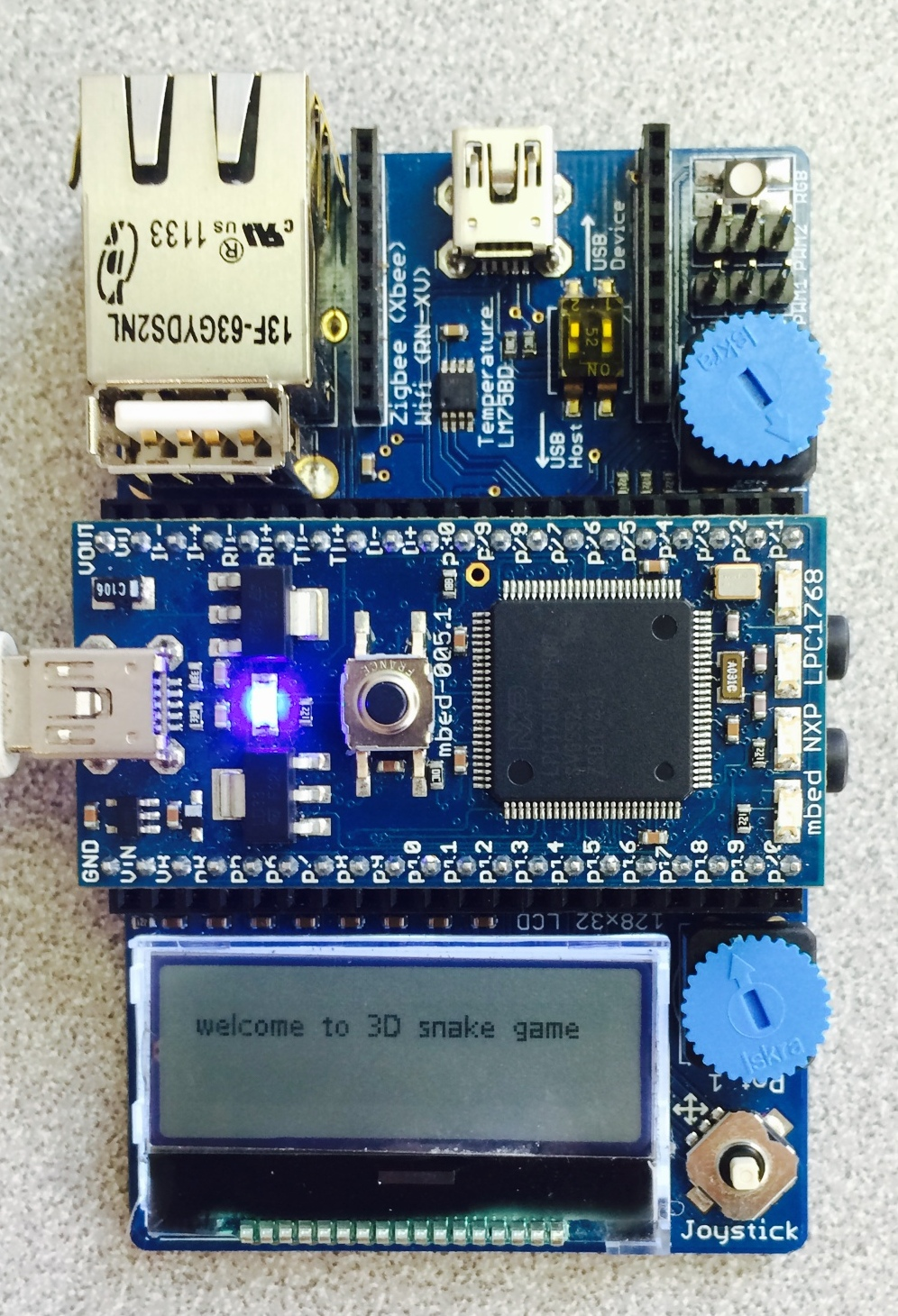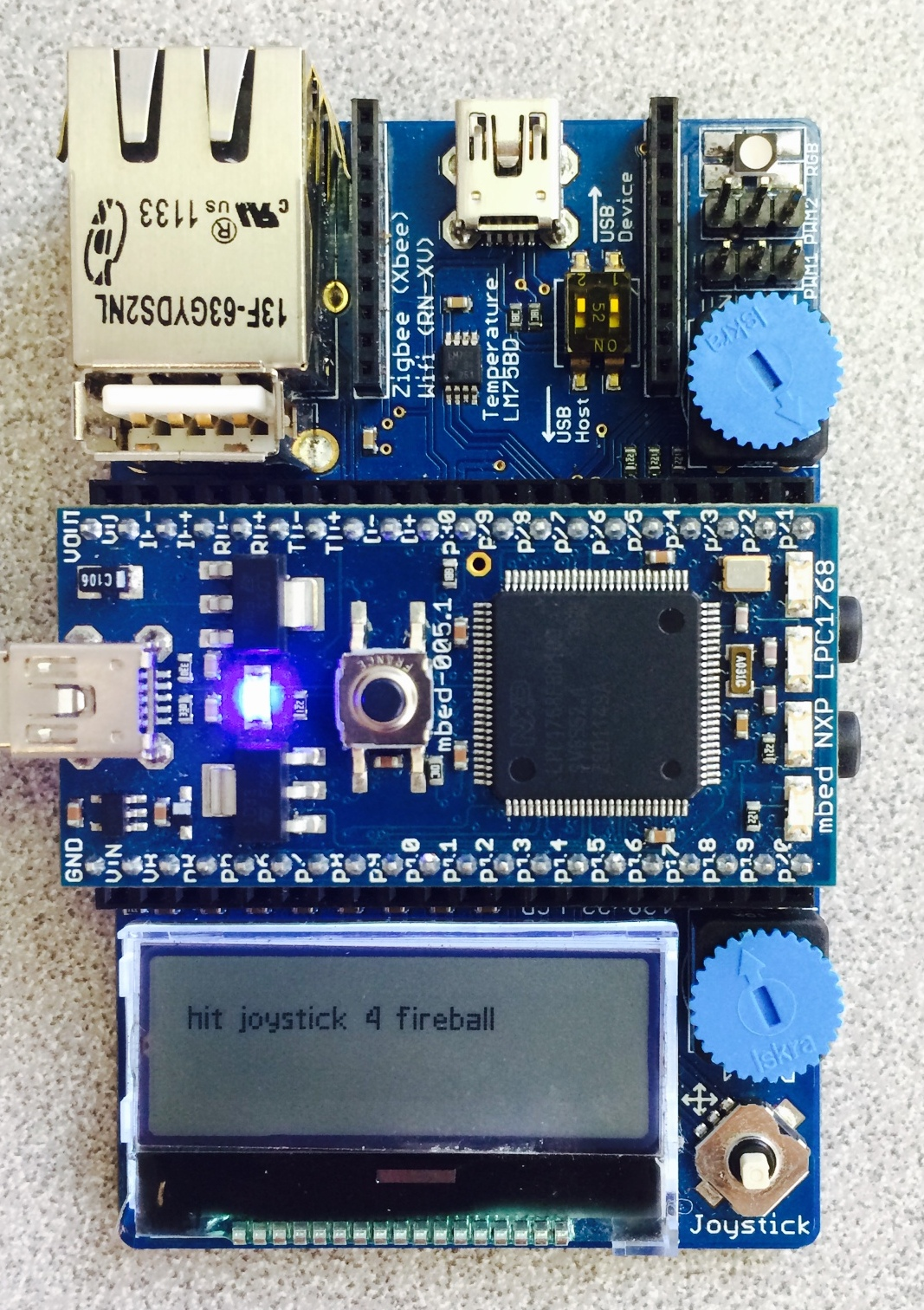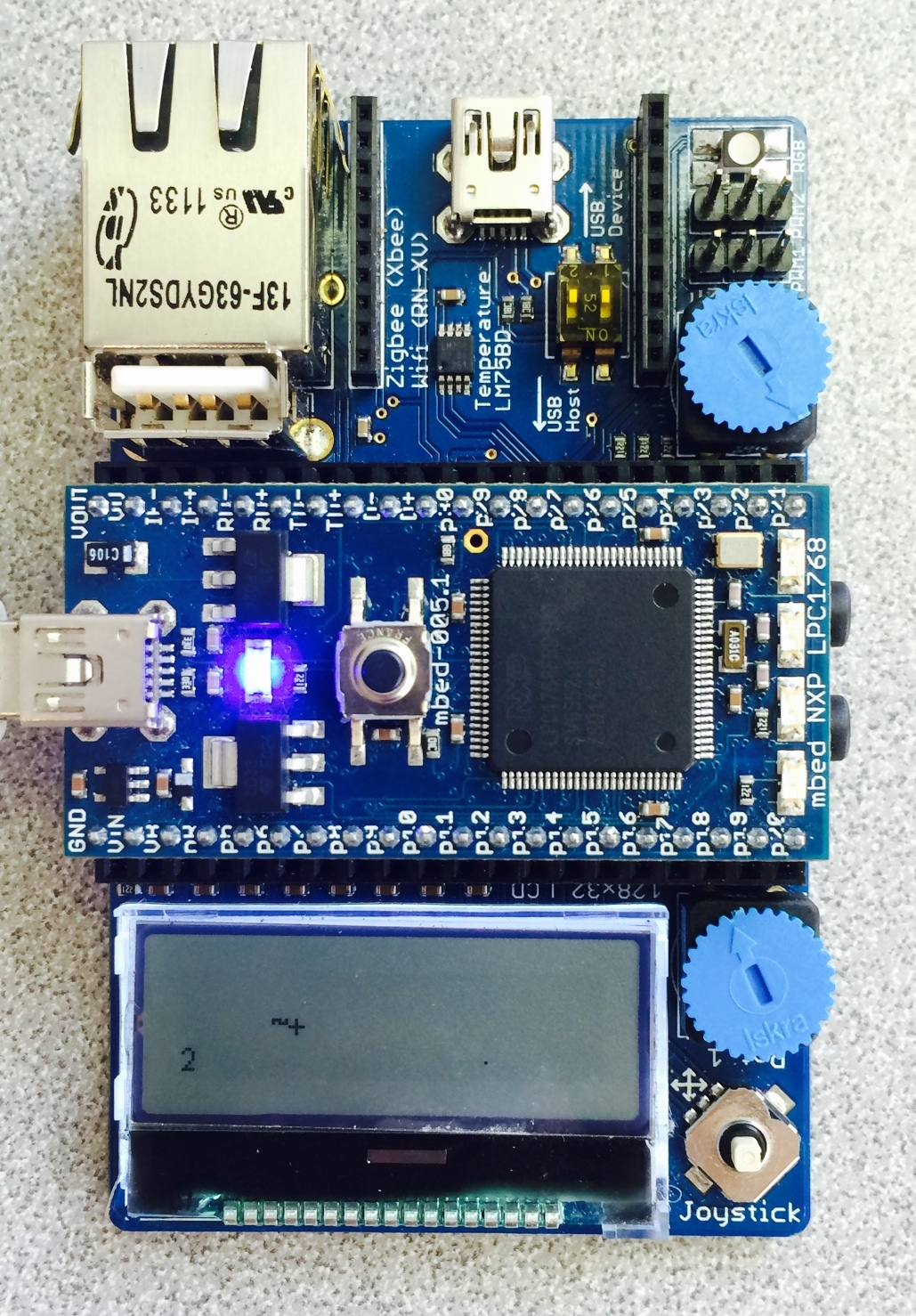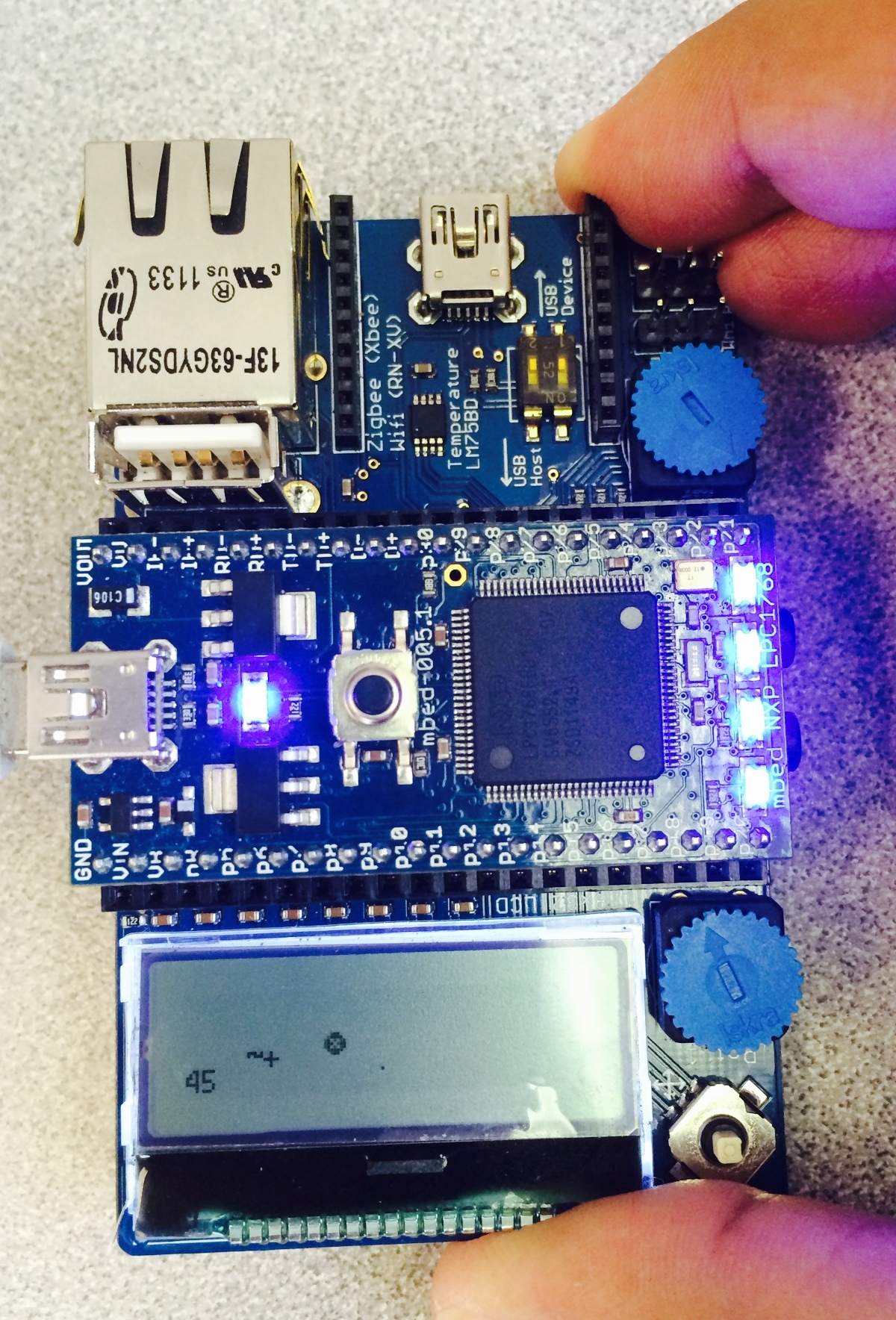# Goal

Our goal with this project is to make a accelerometer driven snakes game for the NXT 1768, using its application board. We wish to use the inbuilt joystick and accelerometer (a bonus feature) to control the snake and collect food.

# How to Play

The user can use the inbuilt joystick to control the snake. The actual fun stuff starts once the user has a score of more than 5. Once this happens, the user can press the joystick button down and use the accelerometer to control a fireball! The fireball has an ability to decimate anything that comes in its path but this power is available for limited time. The faster to get the food, the more bonus you get.# Process

For accomplishing the same, our first job was to figure out what libraries were needed to get the required functionality. We ended up finding that we needed the following:

• The official mbed library
• C12832 library for the application board LCD
• MMA7760 library for the accelerometer
• time library
• stdlib library

### NOTE

For the joystick, we ended up using a BusIn instead of any of the libraries, as it was easier to implement this way.

#### Import programSnakeGame

3-D snake game for the mbed application board.

### Initialization

```Serial pc(USBTX, USBRX);
C12832 lcd(p5, p7, p6, p8, p11);
BusIn joy(p15,p12,p13,p16);
DigitalIn fire(p14);
BusOut leds(LED1,LED2,LED3,LED4);
MMA7660 MMA(p28, p27); //I2C Accelerometer
DigitalOut connectionLed(LED1);//Accel OK LED
```

### Important variable declarations

```srand(time(NULL));
int r = rand();
int i=0;
int dimX = 120;
int dimY = 28;
int x=0,y=0;
lcd.cls();
lcd.locate(0,3);
lcd.printf("welcome to 3D snake game");
wait(2);
lcd.cls();
lcd.locate(0, 3);
lcd.printf("hit joystick 4 fireball");
wait(1.5);
int j = 0;
int cst = 4;
int fDimX = dimX - cst;
int fDimY = dimY - cst;
int flag = 0;
int p1 = r % fDimX;
int p2 = r % fDimY;
int score = 0;
int bonus = 0;
```

### The WHILE loop

```    while(1) {
//CONDITION FOR CHECKING FOR THE FIREBALL
if (fire && score>5) {
leds=0xf;
flag = 100;
if(bonus == 0){
score -= 2;
bonus = 1;
}
}
// CONDITIONS TO CHECK FOR JOYSTICK INPUT
else {
if (flag == 0){
leds=joy;
// moving the snake backwards
if (joy == 0x4 ){
i= i - cst;
if (i < 0)
i = dimX;
}
// moving the snake forward
else if (joy == 0x8){
i = (i + cst)%dimX;
}
// moving the snake up
else if (joy == 0x1){
j = j - cst;
if (j < 0)
j = dimY;
}
// moving the snake down
else if (joy == 0x2){
j = (j + cst)%dimY;
}
}
// LOGIC FOR THE FIREBALL
if (flag >= 1){
x = (x + MMA.x() * 32.0)/1.5;
y = (y -(MMA.y() * 16.0))/1.5;
lcd.fillcircle(x+63, y+15, 3, 1); //draw bubble
//lcd.circle(63, 15, 8, 1);
wait(.1); //time delay
pc.printf(" score %d", score);
flag -=1;
if (abs(x + 63 - p1) <=cst && abs(y + 15 - p2) <=cst+1){
score +=15 * flag/25;
flag = -1;
}
if (flag < 0)
flag = 0;
bonus = 0;
lcd.fillcircle(x+63, y+15, 3, 0); //erase bubble
}
}
if (flag == 0){
//printing the snake, food and score on the LCD
lcd.cls();
lcd.locate(i,j);
lcd.printf("~+");
lcd.locate(0, 22);
lcd.printf("%d", score);
//pc.printf("the dot is at %d %d\n \r", p1, p2);
pc.printf("snake location %d %d\n \r", i, j);
lcd.locate(p1, p2);
lcd.printf(".");
// CONDITION FOR CHECKING THE SNAKE FOOD COLLISION
if (abs(i + 8 - p1) <=cst && abs(j - p2) <=cst+1){
//pc.printf("the snake is at %d %d\n \r", i, j);
//pc.printf("the dot is at %d %d\n \r", p1, p2);
score = score + 1;
//finding a new random location for food
r = rand();
p1 = r%fDimX;
p2 = r%fDimY;
//boundary checking
if (p1 < 10)
p1 = 10;
if (p2 < 10)
p2 = 10;
lcd.printf(".");
}
wait(0.3);
}
}
}
```

# Result

## Connections

There are no special connections required for this game to work. Just take the mbed 1768 and plug it into a application board and you are ready to go.### Normal Play## Fireball in Action

The fireball is controlled by the accelerometer and it eats up whatever comes in its path. The more you eat and the faster you eat, the more bonus you get.